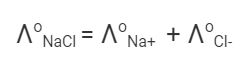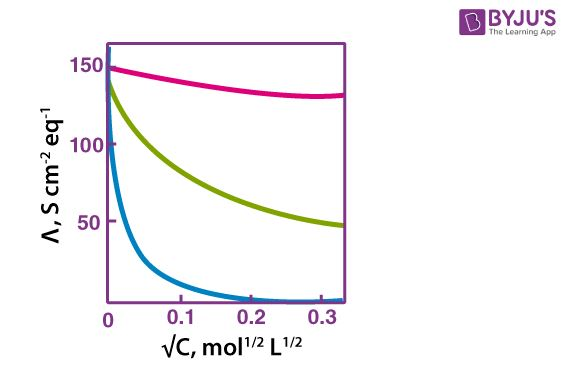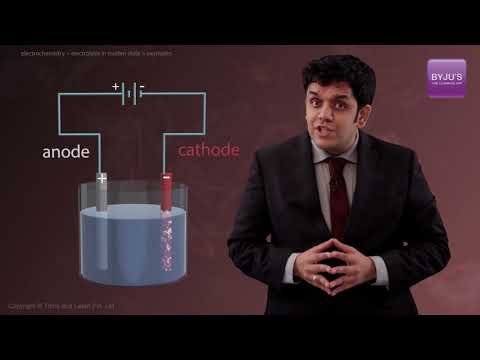# Kohlrausch Law

Kohlrausch Law refers to an electrolyte’s limiting molar conductivity to its constituent ions. It indicates that an electrolyte’s limiting molar conductivity is equal to the sum of the individual limiting molar conductivities of the cations and anions that make up the electrolyte.

The Kohlrausch Law of Independent Migration is another name for this law. The Kohlrausch law and its applications are crucial in the study of dilute liquids as well as electrochemical cells. Among other essential uses, this law is utilised to determine the limiting conductivity of a weak electrolyte.

## What is Kohlrausch’s Law?

Kohlrausch’s law states that the equivalent conductivity of an electrolyte at infinite dilution is equal to the sum of the conductances of the anions and cations.

The molar conductivity of a solution at a given concentration is the conductance of the volume of solution containing one mole of electrolyte kept between two electrodes with the unit area of cross-section and distance of unit length. The molar conductivity of a solution increases with the decrease in concentration. This increase in molar conductivity is because of the increase in the total volume containing one mole of the electrolyte. When the concentration of the electrolyte approaches zero, the molar conductivity is known as limiting molar conductivity, Ëm°.

Kohlrausch observed certain regularities while comparing the values of limiting molar conductivities of some strong electrolytes. On the basis of his observations, Kohlrausch proposed “limiting molar conductivity of an electrolyte can be represented as the sum of the individual contributions of the anions and cations of the electrolyte”. This law is popularly known as Kohlrausch law of independent migration of ions. For example, limiting molar conductivity, Λ of sodium chloride can be determined with the knowledge of limiting molar conductivities of sodium ion and chloride ion.Some important applications of Kohlrausch law of independent migration of ions are:

1. Kohlrausch law helps us in the determination of limiting molar conductivities for any electrolyte. Weak electrolytes have lower molar conductivities and lower degree of dissociation at higher concentrations. The graph plotted between molar conductivity and c1/2 (where c is the concentration) is not a straight line for weak electrolytes. The molar conductivity of weak electrolyte increases steeply at lower concentrations. Therefore, limiting molar conductivity, Λ cannot be obtained by extrapolation of molar conductivity to zero concentration. Hence, we use the Kohlrausch law of independent migration of ions for the determination of limiting molar conductivity, Λ for weak electrolytes.1. Kohlrausch law also helps us in determining the value of dissociation constant from the value of molar conductivity and limiting molar conductivity for a weak electrolyte at a given concentration.

$$\begin{array}{l}α = \frac {Λ}{{Ë_m}^\circ} \end{array}$$

Where,

α = dissociation constant

Λ = molar conductivity

Ëm° = limiting molar conductivity

## Uses of Kohlrausch’s law

• Calculation of Degree of dissociation
• Calculation of solubility of sparingly soluble salt
• Calculation of Dissociation Constant for weak electrolytes
• Calculation of Molar Conductivity for weak electrolytes at infinite dilution

## Electrolysis in Molten State## Frequently Asked Questions – FAQs

### Who discovered the law of independent migration of ions?

This law was discovered by Friedrich Kohlrausch after observing experimental data on conductivities of various electrolytes.

### What is kohlrausch law and its applications?

It’s used to determine an electrolyte’s dissociation constant. It’s used to find out what a weak electrolyte’s limiting molar conductivity is. This law can also be used to determine the degrees of dissociation of weak electrolytes.

### What is Kohlrausch law of independent migration?

According to Kohlrausch’s law of independent ion movement, the limiting molar conductivity of an electrolyte can be described as the sum individual contributions of its cations and anions. Since fewer ions are present for conduction, a solution’s conductivity decreases with dilution.

### Why do we need Kohlrausch law?

The Kohlrausch law can be used to calculate the limiting molar conductivities of any electrolyte. At larger concentrations, weak electrolytes have lower molar conductivities and a lower degree of dissociation.

### What is infinite dilution in electrochemistry?

Infinite dilution is a state of dilution in which the concentration does not change when more solvent is added. In chemistry, the concept of infinite dilution is used to investigate how compounds dissolve in different solvents.

Test your Knowledge on kohlrausch law!

#### 1 Comment

1. Rinkal Kanara

I wanna learn chemistry with byjus, It’s great honor for me to part of your site. Thank you❤.